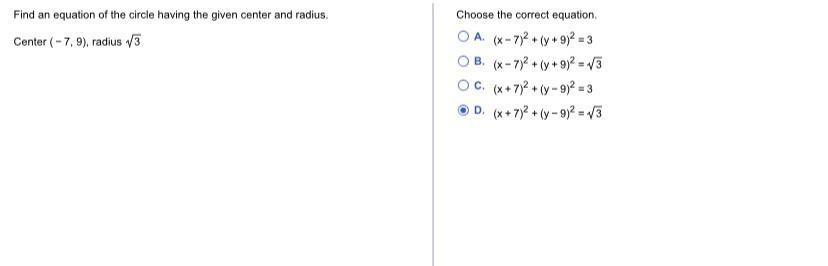Question:

# Find an equation of the circle having the given center and radius. Center (-7, 9), radius 13 Choose the correct equation OA. (x-Find an equation of the circle having the given center and radius. Center (-7, 9), radius 13 Choose the correct equation OA. (x-7)2 + (y + 9)2 = 3 OB. (x-7)2 + (y + 9)2 = 3 OC. (*+7)2 + (-9)2 = 3 OD (+ 7) + (- 9)2 = 13